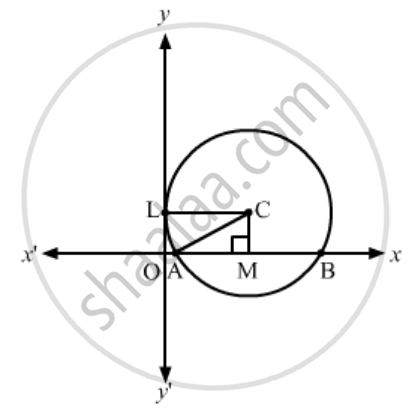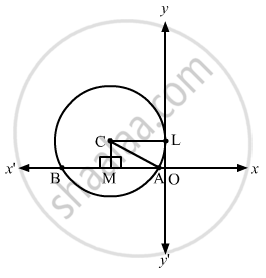Advertisement Remove all ads

# Find the Equations of the Circles Touching Y-axis at (0, 3) and Making an Intercept of 8 Units on the X-axis. - Mathematics

Find the equations of the circles touching y-axis at (0, 3) and making an intercept of 8 units on the X-axis.

Advertisement Remove all ads

#### Solution

Case I: The centre lies in first quadrant.Let the required equation be

$\left( x - h \right)^2 + \left( y - k \right)^2 = a^2$
Here, AB = 8 units and L (0, 3)
In $\bigtriangleup$CAM:
$\Rightarrow C A^2 = C M^2 + A M^2$
$\Rightarrow C A^2 = 3^2 + 4^2$
$\Rightarrow CA = 5$
$\Rightarrow CL = CA = 5$
∴ Coordinates of the centre = $\left( 5, 3 \right)$
And, radius of the circle = 5
$\left( x - 5 \right)^2 + \left( y - 3 \right)^2 = 25$
$x^2 + y^2 - 10x - 6y = - 9$
Case II: The centre lies in the second quadrant.Coordinates of the centre = $\left( - 5, 3 \right)$
And, radius of the circle= 5
$\left( x + 5 \right)^2 + \left( y - 3 \right)^2 = 25$
$x^2 + y^2 + 10x - 6y = - 9$
Hence, the equation of the required circle is
$\left( x \pm 5 \right)^2 + \left( y - 3 \right)^2 = 25$
$x^2 + y^2 \pm 10x - 6y = - 9$
Concept: Circle - Standard Equation of a Circle
Is there an error in this question or solution?
Advertisement Remove all ads

#### APPEARS IN

RD Sharma Class 11 Mathematics Textbook
Chapter 24 The circle
Exercise 24.1 | Q 12 | Page 21
Advertisement Remove all ads
Advertisement Remove all ads
Share
Notifications

View all notifications

Forgot password?